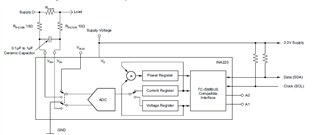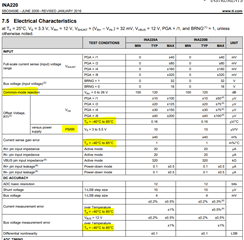If you have a related question, please click the "Ask a related question" button in the top right corner. The newly created question will be automatically linked to this question.

# INA 220 ADC power supply source

Part Number: INA220

INA220 has an ADC block to receive the shunt voltage drop for current measurement

Kindly clarify the following:

What is the power source for this ADC ?    3.3 V  or Bus voltage ?

Does this power source variation affect ADC accuracy ?

Does the variation in ambient temperature affects the ADC performance / current measurement accuracy?

IC block diagram• Hi Shekar,

the ADC is powered from the supply voltage pin "VS" and not from the bus voltage "VBUS".

The effect of supply voltage changes on accuracy of ADC is given in the PSRR specification in the table of section 7.5. The effect of temperature changes is given at four places in section 7.5, "offset voltage" versus temperature, "current gain sense error" versus temperature, "current measurement error" versus temperature and "bus voltage measurement error" versus temperature.

Kai

• Hello Shekhar,

Kai is correct. The ADC is powered off the voltage as Vs pin. All level shifting of the shunt voltage from VCM to ground level for internal ADC is done internally. All error effects from power supply (PSRR), VCM (CMRR), and temperature are characterized and specified in the electrical specification table.You can learn about current sense amplifier/power monitors in our free video series here. Videos cover error sources and total error calculations.

https://training.ti.com/ti-precision-labs-current-sense-amplifiers-introduction-different-error-sources?context=1139747-1139745-1138708-1139852-1139849

Here is another potentially helpful document on understanding how to configure our digital devices:

https://www.ti.com/lit/an/sboa511/sboa511.pdf?ts=1625076381521&ref_url=https%253A%252F%252Fwww.ti.com%252Fproduct%252FINA220

Hope this helps.

Best,

Peter

• Hi , If VS is the power supply for ADC, Is there any internal reference voltage used for ADC (Since Vs may vary  ~ 3.2V to 3.5V , Does it affect the Voltage & current accuracy)

• Hi , If VS is the power supply for ADC, Is there any internal reference voltage used for ADC (Since Vs may vary  ~ 3.2V to 3.5V , Does it affect the Voltage & current accuracy)

• Hey Shekhar,

I am not sure if the the internal ADC has an internal reference voltage, but it probably does. However, what this reference is or how it is generated from VS is moot.

In order to calculate the error caused by a varying Vs voltage, you simply refer to the PSRR specification (10uV/V) to calculate the input offset voltage (Vos_psrr) generated at the two different Vs levels.

If Vs = 3.2V, then Vos_psrr = ± |3.2V-3.3V|*10uV/V = ± 1 uV.

If Vs = 3.5V, then Vos_psrr = ± |3.5V-3.3V|*10uV/V = ± 2 uV.

To calculate total input offset voltage at any point in time, add up all of the individual sources of offset error:

Vos_total = Vos + Vos_psrr + vos_cmrr + Vos_tempDrift

Where Vshunt dynamic relative offset error is calculated as:

eVos = 100*Vos_total/Vshunt

---

Now the following exercise above applies to slow DC shift in VS (<10 Hz). If Vs is varying like an AC voltage from 3.2V to 3.5V with some  higher frequency, then the error could be much higher depending on the frequency. Unfortunately, there is not AC PSRR plot in the datasheet, but if the VS voltage is noisy from 3.2V to 3.5V, then it is highly recommended to add more decoupling capacitance/low-pass filtering to keep this voltage stable at INA220 Vs pin to INA220 GND pin.

Hope this all makes sense.

All of the error calculations are shown in detail with our free training videos:https://training.ti.com/ti-precision-labs-current-sense-amplifiers-introduction-different-error-sources?context=1139747-1139745-1138708-1139852-1139849

Sincerely,

Peter